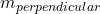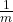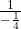## What is the slope of a line that is perpendicular to the line y = –1/4 x + 5

Question

What is the slope of a line that is perpendicular to the line y = –1/4 x + 5

in progress 0
2 weeks 2021-08-29T19:38:22+00:00 2 Answers 0 views 0

slope = 4

Step-by-step explanation:

The equation of a line in slope- intercept form is

y = mx + c ( m is the slope and c the y- intercept )

y = –x + 5 ← is in slope- intercept form

with slope m = –Given a line with slope m then the slope of a line perpendicular to it is= –= –= 4

4

Step-by-step explanation:

To find the slope of a line that is perpendicular to another line simply flip the slope ( so if it is 1/3 flip it to 3 or if it’s 3 flip it to 1/3) and change the sign ( so if it’s positive change it to negative vise versa )

The equation we are given has a slope of -1/4

So flip the fraction ( we would get -4)

And change the sign ( we would get 4)

So the slope of a line that is perpendicular to y = -1/4x + 5 is 4